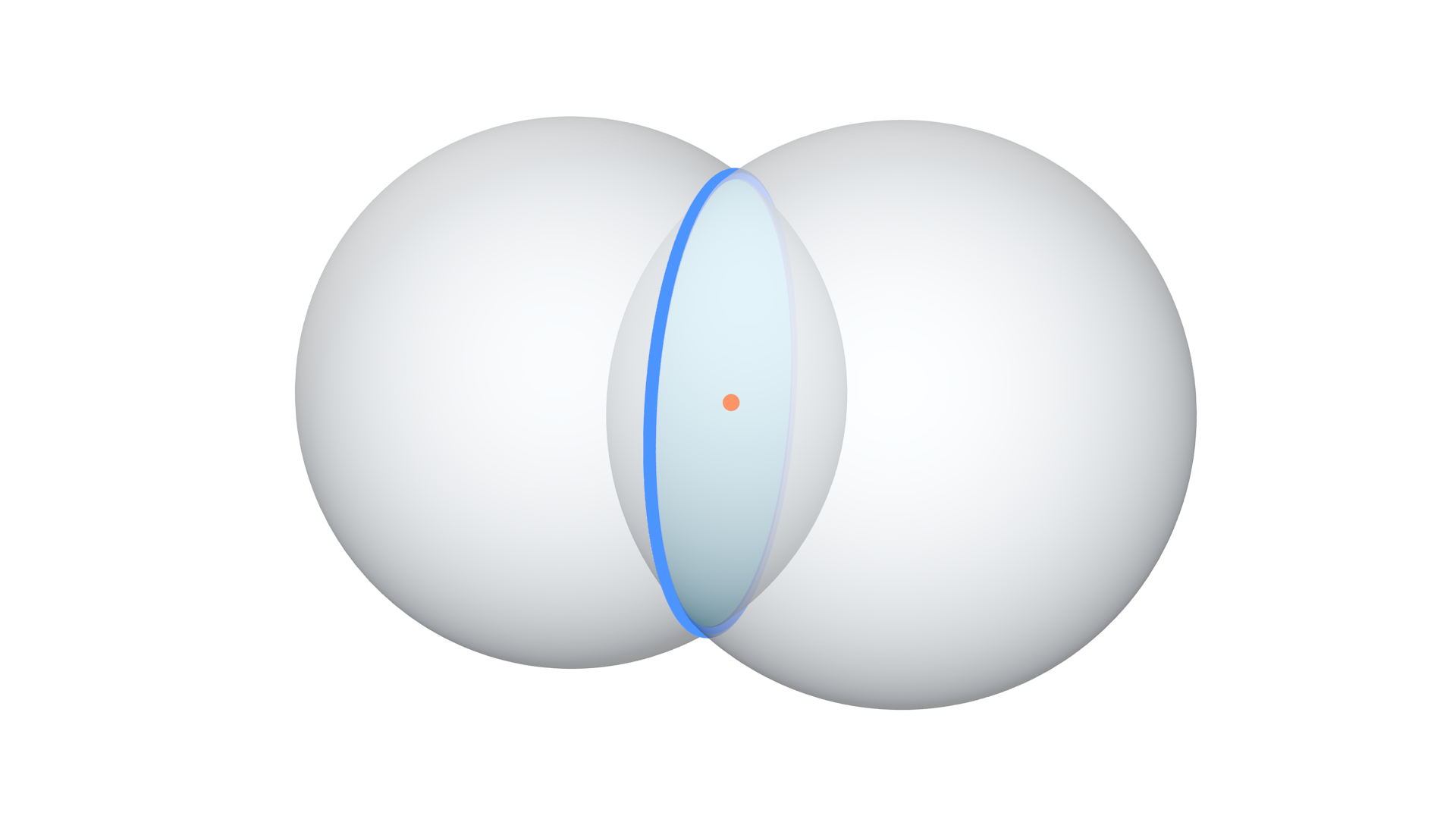# Intersect Sphere Sphere

## Description

This node returns the intersection circle of the two input spheres. A sphere intersects another sphere in a circle, the node returns the radius, normal and center of the intersection circle.

## IllustrationOrange point is the center of the blue intersected circle.

## Inputs

• Sphere 1 Center - The location of the center of the first required sphere.
• Sphere 1 Radius - The radius length of the first required sphere.
• Sphere 2 Center - The location of the center of the second required sphere.
• Sphere 2 Radius - The radius length of the second required sphere.

## Outputs

• Circle Center - The location of the center of the intersected circle.
• Circle Radius - The radius length of the intersected circle. If this values is zero and Is Valid is True, that means that the spheres are touching at the Circle Center.
• Is Valid - A boolean which is True if an intersection was found, and False otherwise.